Subsections

## 5.1.3 Transconductance Study

One of the difficulties, which still hampers the wide use of HEMTs, is the very strong dependence of the transconductance on the gate voltage. As the derivatives of the transconductance with respect to the gate voltage are detrimental to intermodulation distortion [384,19], a profound knowledge of the causes for the transconductance nonlinearity significantly helps the selection of a proper load resistance. The problem has been addressed in numerous works, e.g. [385,386]. Interface roughness and hot-phonon scattering have been ruled out as possible reasons for the strong dependence of the transconductance on gate voltage. While real space transfer effects are relevant in GaAs-based pHEMTs , the lack of a significant leakage current in the studied structures counts against it. Recently, the decrease of transconductance has been attributed to the strong nonlinearity of the source-gate resistance. This has been shown in a couple of studies relying on experimental measurements combined with simulations [385,386], which employ specially tailored carrier velocity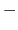electric field characteristics. Here, a good agreement with experimental data is achieved by using electron mobility Model B based on Monte Carlo simulation results. The electron transport in the source-gate and gate regions is studied in a wide range of gate-source voltages and the impact of scaling the source-gate distance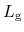on transistor performance is discussed . It is shown that the transconductance decrease should not be attributed to negative differential mobility effects, which is also reproduced by using a velocity-field characteristics from MC simulation results. Device C is used for the study with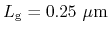, which has been already described in the previous sections.

### 5.1.3.1 Simulation Results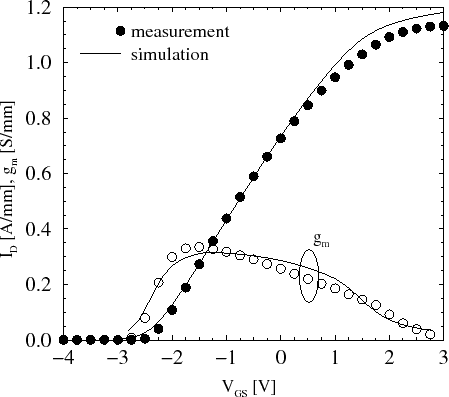Fig. 5.25 shows the measured and simulated transfer characteristics of the device. Without any changes in the models or model parameters a very good agreement is achieved for the drain current as well as for the transconductance. The simulated transconductance exhibits roughly the same maximum value as the measurement and adequately follows the decrease at higher gate voltage. In order to gain a better understanding of the carrier transport process in the device, the transconductance can be expressed as: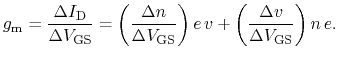(5.1)

The first term describes the contribution of the change in carrier concentration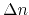. The simulations show that it is substantial only under the gate, as in the source-gate and gate-drain areas no variation of the carrier concentration with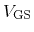is observed. The rapid increase in concentration in the bias range near the maximum transconductance combined with a high electron velocity (Fig. 5.26) indeed results in some contribution of this term to the overall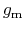. However, this contribution is limited to a small area under the gate in a narrow voltage range, therefore for the further studies we focus primarily on the second term (the change in carrier velocity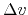).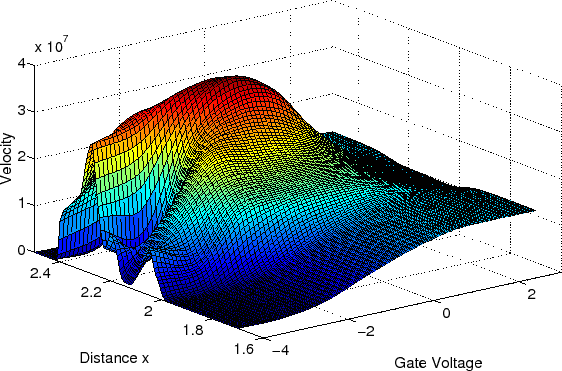Fig. 5.26 shows the velocity along the channel of the device for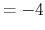V to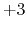V (the gate is located from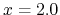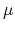m to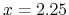m). There are two distinguishable regions: the source-gate region and the effective gate region (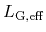). The latter exhibits a high velocity up to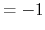V, which then decreases abruptly. This is to be attributed entirely to the electric field profile, which is depicted in Fig. 5.27. The complex form at lowis due to the negative differential velocity at high electric fields, for which the mobility model accounts. As the channel under the gate is entirely depleted at this bias, there is no notable effect on the DC characteristics of the device. The flat distribution of the product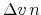(second term in (5.1)) in the gate region as shown in Fig. 5.28 confirms this reasoning.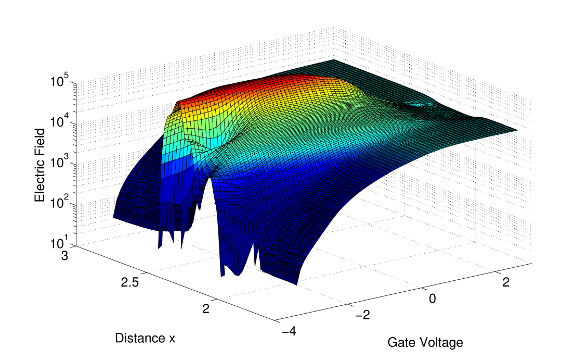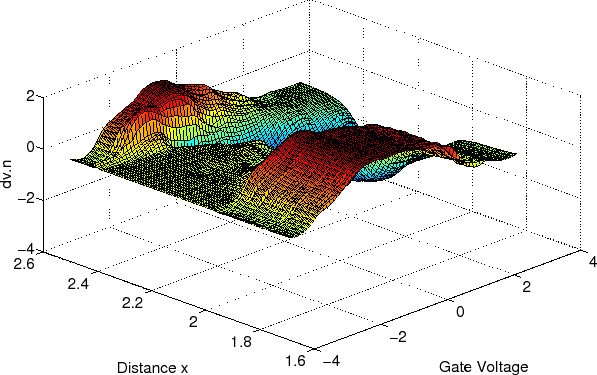In the source-gate region a steady increase of the velocity is observed between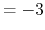V and 0 V, which corresponds to the increase in the electric field. Notably, the electron velocity is very low forV and almost constant for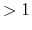V. The resulting productshows a distribution which is very similar to the transconductance characteristics. The decrease of the electric field and, consequently, of the electron velocity under the gate at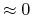V produces a significant negative region. Furthermore, the electron mobility in the source-gate region decreases significantly with higher, which results in the higher source-gate resistance.

Based on these observations several conclusions are self-evident:

• The electron velocity at low electric fields in the source-gate region has the highest impact on the transconductance.
• The decrease of transconductance is caused by the quasi-saturation of the electron velocity in the source-gate region, due to the reached maximum electric field in this region.
• At very high gate bias the electric field reduces further the velocity under the gate and causes the secondary collapse of the transconductance.

### 5.1.3.2 Source-Gate Distance Scaling

Some authors have pointed out the positive effects of downscaling the source-gate distance (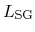) on the transconductance [386,388]. Based on the structure described above, four more devices are introduced, which share the same geometry except for the source-gate distance: one device with a source-gate distance 0.2m longer than the nominal value (+0.2m), and three devices with source-gate distances 0.20.6m shorter than the nominal value (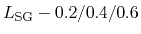m). In agreement with previous works, decreasing the source-gate distance results in a higher peak transconductance (Fig. 5.29). Also with shorter distance a flatter peak is achieved. These improvements are, however, at the cost of a transconductance collapse at lower. In order to find the source of this effect the impact of the carrier velocity and concentration is studied separately as in (5.1).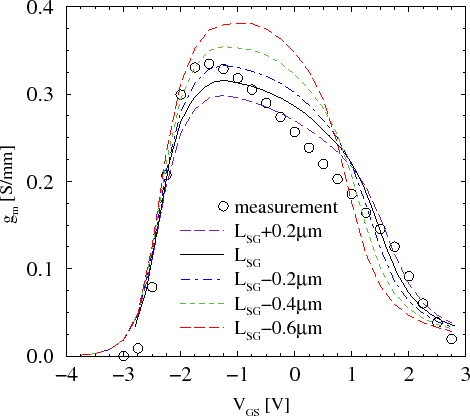The simulations show that the change of carrier concentration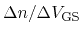is roughly equal for devices with shorter, thus the different transconductance characteristics are due to the different change of the electron velocity with gate bias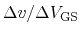. Fig. 5.30 shows the velocity change in the real device (nominal) and a device with a0.6m shorter for two gate voltagesV and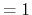V. The former corresponds to the peak, in which the transconductance of the shorter device is higher. The reason is the higherin the source-gate region of the smaller device due to the considerably higher electric field. In the second point (=1 V)in the shorter device is lower overall, causing the lower. It must be noted thatis lower not only in the source-gate region due to reaching the maximum velocity earlier, but also in the region under the gate. There the electric field decreases more rapidly in the shorter structure, which results in the lower value of.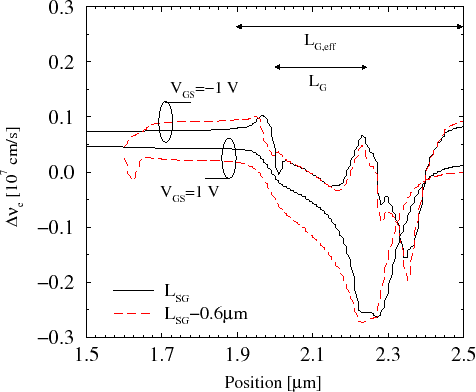These results show that the transconductance can be extensively tailored by appropriate scaling of the source-gate distance. However, down-scaling ofis limited by breakdown effects. Contrary to other studies, here the introduction of a channel implantation or a n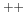cap layer is not supported, as the higher donor concentration deteriorates the electron mobility. Last, it is shown that with a carefully calibrated setup various effects can be successfully explored.

S. Vitanov: Simulation of High Electron Mobility Transistors